You are given a string and a set of characters. Your task is to return any shortest substring that contains all the characters in the set.

Consider string 697581539 and the set of characters {1,5,9}. The only possible answer is 1539 as illustrated in the following diagram.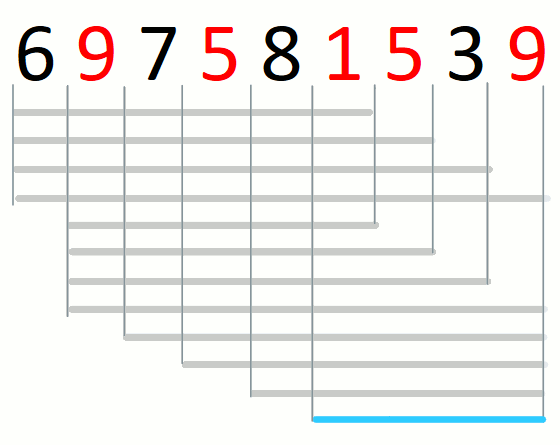When there is no shortest substring, return the empty string.

Input. The input consists of one or more cases. Each case consists of two strings, each one on a separate line. The first string is the string where you will search for a shortest substring. The second string indicates the characters in the set. The input is terminated by EOF. The following is a sample input file.

Output. For each input case, print on a single line a shortest substring or the empty string. The following is the output file that corresponds to the sample input file.

# Problem Structure

Given an input pair of set and string, the structure of the string consists of solutions, candidate solutions, and pre-candidate solutions.

A pre-candidate solution is a substring that contains all characters in the set. For example, consider the pre-candidate solutions for string 697581539 and set {1,5,9}.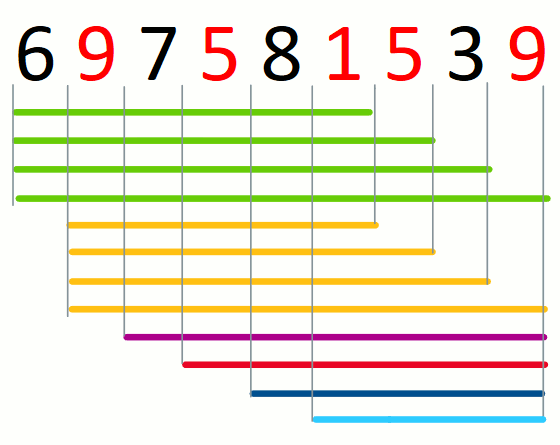For a given prefix of the input string, a candidate solution is the suffix that is a pre-candidate and shortest. There may not be a candidate solution for a given prefix. For example. consider the candidate solutions for string 697581539 and set {1,5,9}.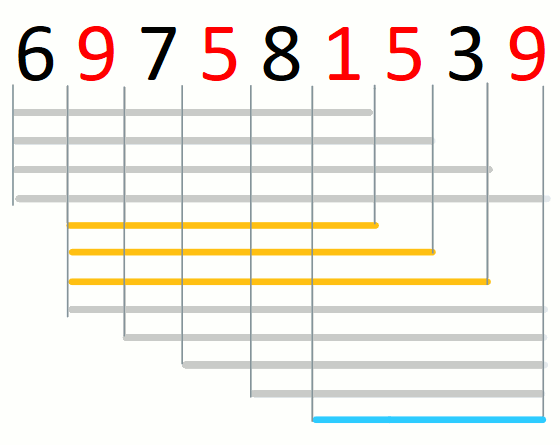A solution is a shortest candidate solution in the string. When there are no candidate solutions, the solution is the empty string. For example, consider the solution for string 697581539 and set {1,5,9}.# Approach

Our approach to the problem consists in finding the leftmost candidate solution (if any) and transforming each candidate solution into the next from left to right (if any). The process involves expanding and contracting a window. The following animation illustrates the approach over string 697581539 and set {1,5,9}.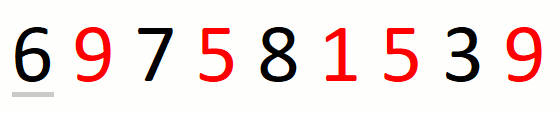Finding the leftmost candidate consists in finding the leftmost pre-candidate and refining it until we have the leftmost candidate. For string 697581539 and set {1,5,9}, this part of the approach is the following.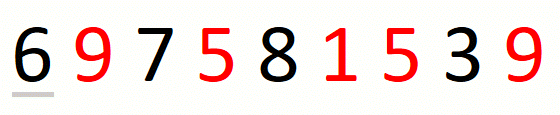Finding the leftmost candidate consists in expanding the window to the right until we cover all characters in the input set. When we do not find all characters in the set, we know that there is no solution and we return the empty string. For string 697581539 and set {1,5,9}, the process is the following.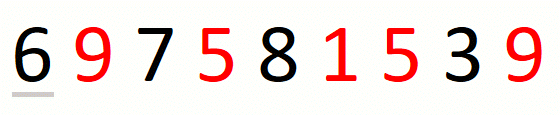Refining the leftmost pre-candidate into the leftmost candidate consists in contracting the window to the right until we cannot drop a character. We cannot drop a character when the character is in the input set and there are no more copies of the character in the window. For string 697581539 and set {1,5,9}, the process is the following.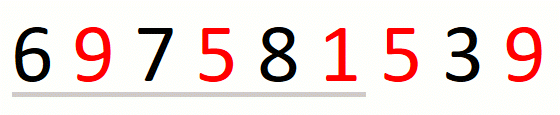We transform a candidate solution into the next by a sequence of expansions and contractions of the window. Every expansion extends the window by one position. A given expansion is not followed by a contraction when we cannot drop the first character in the window. A given contraction drops characters until we cannot do it anymore. This part of the approach is the following.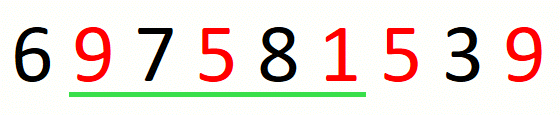We update the current solution after every contraction of the window.

# Implementation

The following is our Ruby implementation of the approach.

Procedure get_initial_right corresponds to finding the first pre-candidate. The main loop of shortest_substring_that_contains_letters corresponds to the rest of the approach. Variables l and r indicate the boundaries of the window.

The crucial aspect of the implementation is counting characters covered by the window. We use dictionary c for that purpose. Dictionary c consists of a counter for each character in the input set.

We use dictionary c in the following way. Procedure get_initial_right counts the characters for the first pre-candidate. When we contract, we use c to never drop a character that we cannot drop. When we contract and we reach a character in the input set, we stop when this is the only copy left in the window (line 39) and we decrease its counter when there is at least another copy (line 42). When we expand and we reach a character in the input set, we increase the counter of the character.

The main loop stops when we cannot expand the window anymore (line 58).

The runtime of our implementation is $O(n)$ where $n$ is the length of the input string. The reason is that the number of iterations of nested loop (lines 37 to 46) is bounded by the length of the input string.